# Subtract Multiple Cells / Columns in Excel & Google Sheets

In this tutorial, you will learn how to subtract cells and columns in Excel and Google Sheets.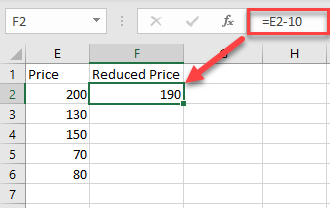## Subtract a Number From Multiple Cells

To subtract a number from a range of cells, click on the cell where you want to display the result, and type “=” (equal) and the cell reference of the first number then “-” (minus) and the number you want to subtract.

In this example, start with cell E2 (200). Cell F2 will show the Price in E2 reduced by 10. So, in cell F2, enter:

``=E2-10``You can then copy this formula down the column to the rows below. To do that, just click on the cell where you entered the formula and place your cursor on the right bottom corner of the cell. When it changes to a plus sign, drag it down to the rest of the rows where you want to apply the formula (in this example, F2:F6).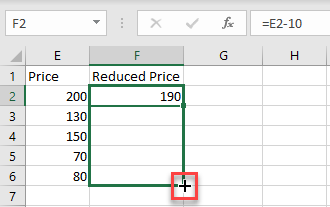As a result of Relative Cell referencing, the constant (in this example, 10) remains the same, but the cell address changes according to the row you are in (so F3=E3-10, and so on).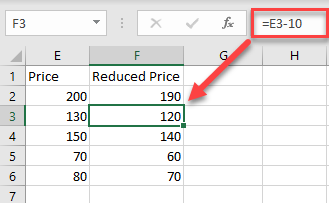## Subtract Multiple Cells With Paste Special

You can also subtract a number from multiple cells, and return the result as a number in the same cell.

1. First, select the cell with the value you want to subtract (in this example, cell G11 ), right-click on it, and from the drop-down menu, choose Copy (or use the shortcut CTRL + C).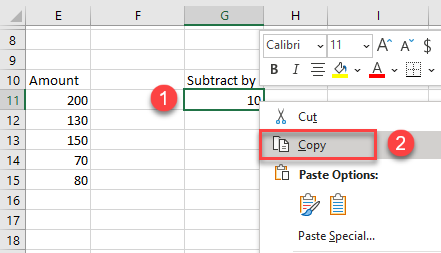2. After that, select the cells where you want to subtract the value and right-click on the data range (in this example, E11:E15). In the drop-down menu, click on Paste Special.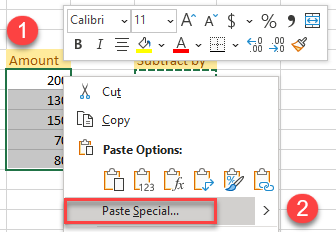3. The Paste Special window will appear. Under the Operation section choose to Subtract, then click OK.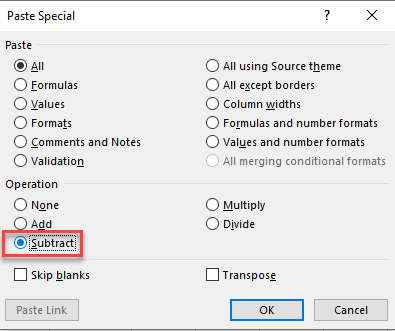After that, you see the result as a number within the same cells. (In this example, 10 was subtracted from each value from the data range E11:E15 .) This method is better if you don’t want to display the results in a new column, because the new (decreased) results are displayed in the original cells.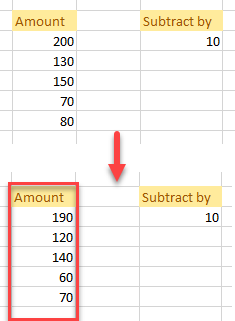## Subtract a Column With Cell References

To subtract an entire column from another using cell references, select the cell where you want to display the result, and enter “=” (equal) and the cell reference for the first number then “-” (minus) and the reference for the cell you want to subtract.

For this example, calculate the difference between Price 1 (A2) and Price 2 (B2). So, in cell C2, enter:

``=A2-B2``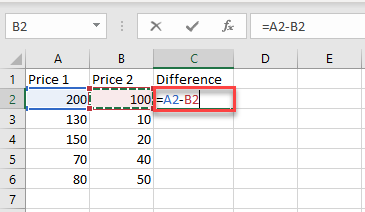To apply the subtractions to an entire column, just copy the formula down to the rest of the rows in the table (in this example, C2:C6).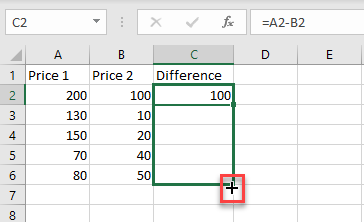Now, you have a new column with the result of the subtraction.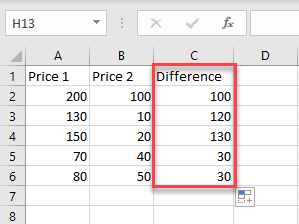Using the methods above, you can also add, multiply, or divide cells and columns in Excel.

## Subtract Cells and Columns in Google Sheets

In Google Sheets, you can subtract multiple cells using formulas in exactly the same way as in Excel. However, you can’t subtract using the Paste Special option.